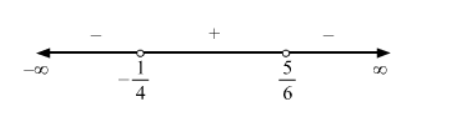# Solve the followingQuestion:

$\frac{6 x-5}{4 x+1}<0$

Solution:

$\frac{6 x-5}{4 x+1}<0$

Equating $6 x-5$ and $4 x+1$ to zero, we obtain $x=\frac{5}{6}$ and $-\frac{1}{4}$ as the critical points.$\therefore x \in\left(\frac{-1}{4}, \frac{5}{6}\right)$